###### UNKNOWN CIVILIZATION OF PAZHAYARU
December 31, 2018

## EFFECT OF TEMPERATURE AND DIAMAGNETIC SUSCEPTIBILITY ON IMPURITY BINDING ENERGY OF A SPHERICAL QUANTUM DOT

#### *A. Rejo Jeice  & **K.S. Joseph Wilson

*Assistant Professor, Department of Physics, Annai Velankannai College, Tholayavattam, India
**Associate Professor, Department of Physics, Arul Anandar College, Karumathur, Madurai, India

### Abstract

The effect of temperature and diamagnetic susceptibiltiyon the hydrogenic donor impurity binding energies of the low-lying states in a GaAs/Ga1-xAlxAsspherical quantum dot is investigated by variational approach within the effective mass approximation. Our result shows that the impurity binding energy decreases with increase in temperature and the diamagnetic susceptibility increases with increase in temperature for larger dot radii and it is not it is not significant to low dot radii. All the calculations have been carried out with finite barriers, and good agreement is obtained with the existing literature values.

Keywords: spherical quantum dot, square well confinement, binging energy, effective mass.

### 1.Introduction

In the recent years the fabrication of semiconductor quantum structures with zero dimension (Quantum dot) will shows excotic electronic behaviour such as the observation of discrete electronic states in GaAs/GaAlAs nanostructures. In which the study of impurities in the semiconductor becomes interesting due to the confinement nature of low dimensional semiconductor systems(LDSS).A doped hydrogenic impurity in a quantum dot influences both the electrical and optical properties because of coulomb interaction between the electron and the impurity ion[2,3]. The addition of impurity in semiconductor QD can change the properties of quantum devices dramatically. There are numerous theoretical works on donor binding energies in LDSS [4-8]. Many of the authors who had studied the donor binding energy with external effects of electric field, magnetic field and hydrostatic pressure. The study of donor binding energy with temperature is found in the following papers.

A.M.Elasby found that the temperature affects the shallow donor states in quantum well super lattices.G.Rezaei et al. found that the donor binding energy which effects the dot radius, potential depth, impurity position, external electric field, conduction band non-parabolicity,hydrostatic pressure and temperature on the hydrogenic impurity in a spherical quantum dot[5,6].Gh.Safarpour et al. studied the binding energy and diamagnetic susceptibility of an on-center hydrogenic donor impurity in a spherical quantum dot placed at the center of the cylindrical nano-wire.R.Khordad calculated the effect of temperature on the binding energy of excited states in a ridge quantum wire. He found that the impurity location play important roles in the binding energy of ground state and two low lying excited impurity states for a V-groove quantum wire . In the previous paper one of the authors had studied the effect of hydrostatic pressure and polaronic mass on the correlation energies in a SQD .

In the present paper, a systematic study of variation temperature and diamagnetic susceptibility of on-centerhydrogenic impurity binding energy of a spherical quantum dot as a function of dot size has been attempted in a finite confinement model. The purpose of the present work is two-fold. The first is to calculate the donor binding energy for ground and excited state for various temperatures. Secondly we calculate the diamagnetic susceptibility of the donor binding energy. The method followed is presented in the section 2 while the results and discussion are provided in section 3.

### 2. Method

#### 2.1. Binding energy of a donor

We consider a single electron in a Spherical quantum dot in the finite barrier model. In the presence of on-center hydrogenic impurity, within the effective mass approximation, the Hamiltonian is given by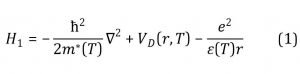where VD(r,T), ε(T) and m*(T) is the confining potential, the static dielectric constant and the effective mass of the electron at the conduction band minimum, which is 0.067m0 for GaAs , where m0 is the free electron mass. In our numerical calculations we use atomic units in which free electron mass, electronic charge and the Planck’s constant have been assumed as unity. The confining potential VD(r) is given by [11,12],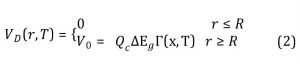where V0 is the barrier height, Qc is the conduction band offset parameter which is taken to be 0.6 . The band gap difference depends of the concentration of Al. In our case Ga1-xAlxAs is the barrier medium in which GaAs dot is embedded. The total energy difference  between the dot and barrier media, as a function of x, is given by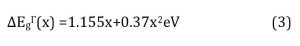In the present work we have chosen x=0.3 and the value of V0 turns to be 227.28meV respectively. The ground state and the excited state eigen functions in the absence of impurity are given by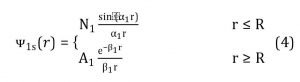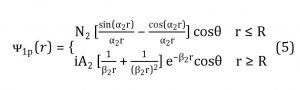where N1 ,N2 ,A1and A2 are normalization constants and α1 and β1 are given by〖 α〗_1=√(2m^* E) and β_1=√(2m^* (V_0-E))

Matching the wave function and their derivatives at the boundary r=R, we get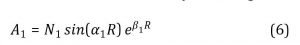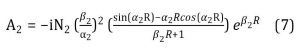The energy eigen values are determined by imposing the Ben Daniel and Duke boundary condition that the normal particle velocity is continuous across the interface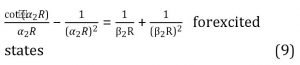Solving these transcendental equations numerically, the confined energies of ground state and excited state are obtained.

When the hydrogenic impurity is placed at the center of the dot, to solve the Schrödinger equation, we choose trial wave function as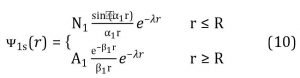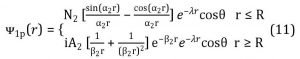where λ is the varational parameter. The ground and excited state energies with the presence of impurity obtained from the expression〉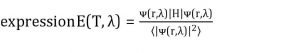This is minimized with respect to varational parameter λ. We obtained the minimum energy Emin(T) by(∂E(T,λ))/∂λ=0. The binding energy of the hydrogenic impurity is defined as the ground energy of the system without impurity present minus the impurity state energy. The ground and excited state donor impurity binding energy isEb(T)=E0-Emin(T).The expression obtained for Emin is too lengthy, hence we refrain from giving them here.

#### 2.2. Effect of Temperature

Due the application of temperature the effective mass, dielectric constant and the barrier height are modified. The total band gap difference between the GaAs and Ga1-xAlxAs barrier medium under the influence of heat is given by [4,16]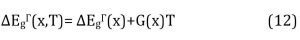Where G(x) = [-(1.15×10-4)] x eV/K. The temperature dependent conduction effective masses of the quantum dot and barrier layer are obtained from the expression [17,18]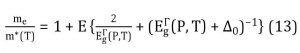where E=7.51eV, is the energy related to the momentum matrix element.Δ0=0.341 eV is the spin-orbit splitting, me is the free electron mass and Ep(T) is the temperature dependent energy gap for the GaAs QD at the τ-point and is given by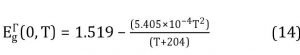The corresponding conduction effective mass in the barrier layer is obtained from a linear interpolation between the GaAs and AlAs compounds’  i.e.,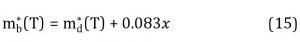where x is the mole fraction of aluminum in the GaAlAs layer. The variation of dielectric constant with temperature is given as,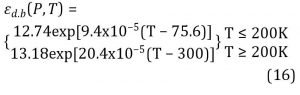The corresponding dielectric constant of Ga1-x AlxAs is given as εb(T)= εd(T)-3.12x. We have calculated the diamagnetic susceptibility of the donor impurity under influence of temperature is given by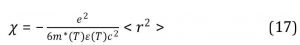where c is the velocity of light and < r^2 > is the mean square distance of the electrons from the nucleus.

### 3. Result and discussion

The results are presented in tables 1-3 and Figs.1-5.The confined energies of the ground state and the excited states are listed in tables 1 and 2.We have computed the effect of temperature on hydrogenic donor binding and diamagnetic susceptibility on a spherical quantum dot.Due the application of the temperature on the SQD the effective mass, dielectric constant and the barrier height of the dot radius are modified. We have noticed that due to the application temperature the effective mass and the barrier height slightly decreases and the dielectric constant increases.

#### Table 1. Confined energies (meV) under temperature in the finite barrier model for ground state

 Dot Radius(Ǻ) T=0K T=100K T=200K T=300K T=400K T=500K 30 212.67 213.38 214.83 216.52 218.34 220.15 35 187.34 188.29 190.19 192.53 195.11 197.86 40 162.55 163.53 165.52 168 170.76 173.74 50 122.56 123.45 125.24 127.48 130.03 132.79 100 41.57 41.95 42.71 43.67 44.77 46.01 150 20.4 20.6 21 21.49 22.08 22.71 200 12.07 12.18 12.43 12.73 13.09 13.47 250 7.95 8.04 8.19 8.41 8.65 8.91 300 5.63 5.7 5.82 5.95 6.13 6.31

#### Table 2. Confined energies (meV) under temperature in the finite barrier model for excited state

 Dot Radius(Ǻ) T=0K T=100K T=200K T=300K T=400K T=500K 50 226.83 – – – – – 55 205.64 206.1 207.75 209.85 212.1 214.27 60 184.46 185.14 187.1 189.6 192.4 195.39 65 165.34 166.56 169.06 172.19 175.7 179.55 100 84.29 85.03 86.56 88.49 90.68 93.1 150 41.64 42.03 42.84 43.86 45.09 46.33 200 24.65 24.9 25.39 26.02 26.72 27.52 250 16.27 16.44 16.77 17.18 17.66 18.2 300 11.54 11.64 11.88 12.18 12.53 12.92

Dashes represent absence of bound states

From the table 1-2 we find that the confined energy decreases as the dot radius increases which is well known in literature [21-22].For a given dot radius as the temperature increases the confined energy also increases which is contract with the application of pressure in the SQD .Thus the pressure effect is opposite to the confined energy. This behavior is due to variation ofmass with the change in confined energy. We also notice that the excited state(1p-state) energies are almost two times the corresponding ground state energies(1s-state) for dot radii greater than 55Å.For smaller dot sizes less than 30Å and 50Å we notice there is no confinement energy for ground and excited states since there is no bound state. These results are contrast to the quantum well case where in there is a bound state for every well size .

#### Fig.1 Variation of confined energy verses Dot Radius in the finite barrier model for ground and excited states.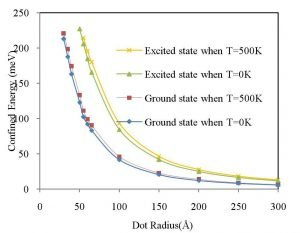Fig.1 represents variation of confined energy for different dot radius of ground and excited states for temperature T=0K and 500K.We notice there is increase in confinement with temperature. At large values of the dot radius the effects of confinement energy on the impurity states become negligible and therefore the energy approaches the corresponding energy of a bulk values. This is in agreement with Ref..

#### Fig.2 Variation of ground state hydrogenic donor binding energy verses Dot Radius in the finite barrier model for a given temperature.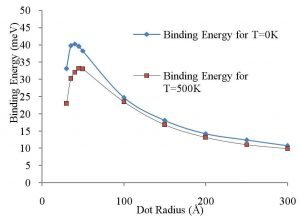Fig.2 shows the variation of ground state hydrogenic donor binding energy verses dot radius for a given temperature. The binding energy increases attains a maximum value and the decreases as the dot radius increases. It is because as the temperature increases the electron is pulled towards one side of the quantum dot resulting the overall decreases of the binding energies.When the dot radius is extremely large, the confining potential has a small influence on the impurity and the wave function approaches to the corresponding state of the free space hydrogen atom.It also seen that the binding energy shows a peak around 40Å, is a well-known feature in a finite well calculation.When the dot radius approaches to infinity the binding energy reaches the three dimensional GaAs, while the dot radius is zero, the binding energy in the barrier region. Hence the confinement becomes negligibly small in the finite barrier problem thus the tunneling becomes huge.Our results are good agreement with Ref..The variation of impurity binding energy occur in smaller dots due to the aluminum concentration. Thus the concentration of aluminum is also important for the SQD. Like other systems when GaAs dot is embedded in Ga1-xAlxAs matrix, the dielectric mismatch becomes insignificant, especially when x is small. The impurity binding energy without considering temperature is about 42 mev for R=50Å .This is in agreement with our result of 38.24 meV.

#### Fig.3 Variation of excited state hydrogenic donor binding energy verses Dot Radius in the finite barrier model for a given temperature.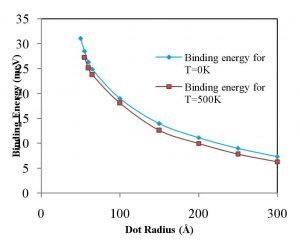Fig.3 shows the variation of excited state(1p-state) hydrogenic donor binding energy verses dot radius for a given temperature. Here we have seen that the binding energy increases by the application of temperature. We notice that the smaller quantum dot shows the greater donor binding energy due to the geometrical confinement. The binding energy in the excited state reaches a maximum at 50 Å in the absence of temperature. At high temperature the impurity binding energy reaches maximum at 55 Å. The effect of temperature has more significant only for smaller dot size.

#### Fig.4 Variation of hydrogenic donor binding energy verses temperature for the ground and excited state.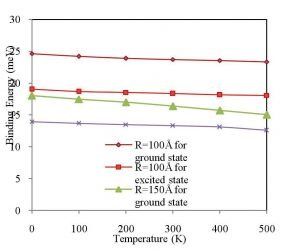Fig.4 we display the variation of hydrogenic donor binding energy with different temperature for the ground and excited state. It have been seen that the temperature increases the donor binding energy decrease linearly. This is contract to the pressure effect in which the pressure increases the donor binding energy increases linearly . It is also seen that the donor binding energy of ground state is higher than of the excited state.

#### Fig.5 Variation of diamagnetic susceptibility verses dot radius in the finite barrier model for a given temperature.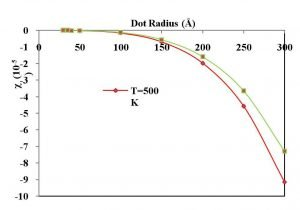Fig.5 we present the variation of diamagnetic susceptibility verses dot radius in the finite barrier model for a given temperature. It is noticed that the variation of susceptibility for the ground state is not pronounced for smaller dot radius due to the domination of geometrical confinement as in Ref. However induced geometrical confinement is dominant beyond 100 Å in which the electron wave function is concentrated more around the impurity ion.Similar results observed in Ref. in which GaN/AlGaN QD in which confinement dominant beyond 90Å.The susceptibility decreases as the dot radius increases. We found that as the temperature is applied it affects mostly on the larger dot radii. As excepted we notice that susceptibility affect slightly to lower dot radii due to the application of temperature. The results are well agreement with Ref..

The important conclusion that emerges from the result of Tables 1-2 and Figures 1-5 is that the temperature effects are important for smaller dots and should be consider in the studies of low dimensional semiconductor systems.

### References

 R.S.Daries Bella and K. Navaneethakrishnan, Solid State Commun. 130 (2004) 773.
 E.Sadeghi and GhRezaie, Parmana Journal of Physics.75 (2010) 749.
 A.John Peter, Physica E, 28 (2005) 225.
 A.M.Elabasy, Physica Scripta, 46 (1992) 473.
 G. Rezaei, N.A.Doostimotlagh and B.Vaseghi, Physica E43 (2011) 1087.
 G. Rezaei, S.F.Taghizadeh and A.A.Enshaeian, Physica E44 (2012) 1566.
 Gh.Safarpour, M.Barati, M.Moradi, S.Davatolhagh, A.Zamani, Superlattices and Microstructures 52 (2012) 387.
 R.Khordad, Physica E 41 (2009) 543
 A.Sivakami, A.RejoJeice, and K.Navaneethakrishnan, International Journal of Modern Physics B.24 (2010) 556.
 O.Madelung Ed., Semiconductor Basic Data, Springer-verlag (1996) 103.
 E. Kasapoglu, H. Sari, I. Sökmen, Physica B 373 (2006) 280.
 G.A. Samara, Physical Review B27 (1983) 3494.
 A R Jeice, Sr G Jayam and K S J Wilson, Indian Journal of Physics 90 (2016) 805.
 A. RejoJeice, and K.Navaneethakrishnan, Brazilian Journal of Physics 39 (2009) 526.
 A.Sivakami and V.Gayathri, Superlattices and Microstructures 58 (2013) 218.
 B.Welber, M.Cardona, C.K.Kim, S.Rodriquez, Physical Review B12 (1975) 5729.
 H.J.Ehrenreich, Journal of Applied Physics 32 (1961) 2155
 E. Kasapoglu, Physics Letters A373 (2008) 140
 D.E.Aspnes, Physical Review B14 (1976) 53319.
 S.Rajashabala and K.Navaneetha krishnan, Superlattices and Microstructures 43 (2008) 247.
 Sr. Gerardin Jeyam and K. Navaneethakrishnan, Solid State Commun. 126 (2003) 681.
 A.Benedictal, B.Sukumar, and K.Navaneethakrishanan, Phys. Stat. Sol.(b) 178 (1993) 167
 S.J.Liang and W.F.Xie.Eur.Phys.J.B81 (2011) 70.
 Zhen-Yan Deng, Jing-Kun Guo and Ting-Rong Lai, J.Phys.Cond.Matter. 6(1994) 5949.
 G. Rezaei, N.A.Doostimotlagh and B.Vaseghi, Commun. Theor. Phys. 56 (2011) 377.
 J.J.Sharkey, Chang Kyoo Yoo and A.John Peter, Superlattices and Microstructure 48 (2010) 248.